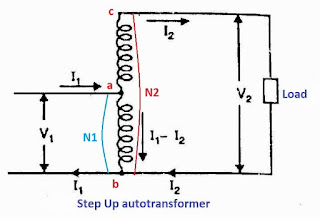Autotransformer is a transformer in which part of the winding is common to both the primary winding circuit and a secondary winding. In normal two winding transformers, primary and secondary winding are electrically isolated but in the case of an autotransformer, the two winding are connected electrically as well as magnetically. Autotransformer also called as variac or variable autotransformer. Autotransformer works as a voltage regulator. With the help of autotransformer, we can get variable voltage at the output.(a) Step down auto transformer

The above diagram (a) shows the connection diagram of step down auto-transformer and figure (b) shows the connection diagram of step-up autotransformer.(b)step-up auto transformer

In both cases step up and step down, the winding "ab" is having N1 turns is primary winding circuit and winding "bc" having Nturns is secondary winding of the auto transformer.

Note that the primary and secondary winding of auto transformer are connected electrically as well as magnetically.

Hence Power from the primary is transferred to the secondary winding conductively as well as Transformer action by mutual induction.

The above diagram depicts the connections of the loaded step-down and step-up autotransformer. In both cases, I1 is the input current and Iis output current, Regardless of Step Up/Stepdown autotransformer, the current in the section of winding that is common to both the primary and secondary is the difference between these two currents I1 and I2. The direction of the current through the common part of the winding depends upon the connection of the autotransformer. because the type of connection decides whether input current I1 or output current I2 is larger.

For a step-down type  I2 > I1 so I2I1 current flows through the common part of the winding. For step Up autotransformer I2 < I1  hence  I1I2 current flows in the common part of the winding.

## Theory of autotransformerAuto transformer diagram

The diagram shows an ideal step down autotransformer with load, hence primary winding 1-3 having N1 turns and secondary winding 2-3 having  N2 turns. The input current is Iwhile output current is shown with I2, in the diagram primary winding portion 1-2 has N1-N2 turns and the voltage across the primary winding part is V1-V2, the current through the common part of the winding is I2- I1  diagram 2 shows an equivalent circuit of the autotransformer.

From the equivalent circuit,

V1I1 = V2I2 (Input apparent power = Output apparent power)

### Output

The primary and secondary windings of autotransformer are connected magnetically as well as electrically, the power transfer from the primary circuit to the secondary is in the form of induction as well as conduction.

Output Apparent power=V2I2

Apparent power transfer by induction=V2(I2 - I1) =V2(I2 - kI2)
=V2I2(1k)=V1I1(1k)
Power transfer inductively = Input x  (1- k)

Power transfer Conductively=I/p- I/p(1- k)
=I/p [ 1- (1-k)]
=I/p x k

## Copper saving in  autotransformer

For same output and voltage transformation, an autotransformer required less copper transformer required less copper than ordinary transformer in first diagram ordinate transformer is depicted and the second diagram shows at a transformer having the same output and voltage transformation ratio k

The length of copper required in autotransformer winding is directly proportional to the turns, and the area of cross-section of the winding wire is proportional to the current rating of auto transformer, therefore, copper required for winding is proportional to the current X turn.

Weight of copper in autotransformer winding ∝ Current x Turn

Winding transformer

Weight of copper required  I1N1 +I2 N2

Autotransformer

Weight of copper required in section 1-2     I1 (N1 - N2)
Weight of copper required in section 2 -3   N2 (I2 - I1)

therefore,
Total weight of Cu required   I1 (N1 - N2) +N2(I2 - I1)
Weight of Cu in autotransformer (Wa) = (1 - K) x Wt. in ordinary transformer (Wo)
Wa = (1 - K) x Wo

Saving in Copper = Wo - Wa = Wa - (1 - K)Wo = K Wo
or
Saving in Copper = K ´ Wt. of Cu in ordinary transformer

Thus if K = 0.1, the saving of Cu is only 10% but if K = 0.9, saving of Cu is 90%. Therefore, the nearer the value of K of autotransformer is to 1, the greater is the saving of Copper.

(1) An autotransformer required less Copper than an ordinary transformer of a similar rating.

(2) It has better voltage regulation and operates at a higher efficiency than a 2-winding transformer of the same rating.

(3) It has a smaller size than an ordinary transformer of the same rating.

(4) An autotransformer requires a smaller exciting current than a 2-winding transformer of the same rating.

It may be noted that these advantages of the autotransformer decrease as the ratio of transformation increases. Therefore, an autotransformer has marked advantages only for relatively low values of transformation ratio

Some disadvantages autotransformer are given as follows

(1) There is a direct connection between the primary winding and the secondary. Therefore, the output is no longer directly isolated from the input.

(2) An autotransformer is not safe for stepping down a high voltage to a low voltage.  in case an open circuit develops in a common part of winding then full primary voltage appears across the load, it will dangerous to both people and equipment.

(3) The short-circuit current is much larger than for the two-winding transformer of the same rating.

## Applications

(i) Autotransformers are used to compensate for voltage drops in transmission lines. When used for this application, these are known as booster transformers.

(ii) It is also used as a reduced voltage starter for the Induction motor.

(iii) Autotransformers are used to obtain a variable supply.

Related.